July. 2022

# Working with Vector Data

In this section, we will use the sf package to work with vector data. We will look at different data formats and work with vector data in

• the .csv,
• the .shp and
• the .gpkg format.

# Working with .csv Files

To read the data, we use the read.csv function and specify the location of the file as well as the separator and define that our file has a header

``````point_pattern_csv <- read.csv("./data/raw_data/random_points.csv",
sep = ',', header = TRUE) ``````

After that, we look at our data, to see if everything works as expected

``head(point_pattern_csv) ``
fid id xcor ycor slope aspect tpi
1 0 27.17486 39.05253 0.2522874 2.435236 -8.2139874
2 1 27.07537 39.00996 0.0698527 3.403179 -3.2234774
3 2 26.99747 39.04333 0.0013852 4.850579 0.0225453
4 3 26.97282 39.03483 0.0919539 5.199027 -6.2441344
5 4 27.01735 39.01458 0.0002953 4.905550 -0.0705314
6 5 26.95903 39.03895 0.0362702 3.079486 -3.0725265

And now we can see, that our point-data has attributes like xcor, ycor, slope, aspect and TPI

# Spatial Object?

If we look at the structure of our data like this,

``str(point_pattern_csv) ``
``````## 'data.frame':    100 obs. of  7 variables:
##  \$ fid   : int  1 2 3 4 5 6 7 8 9 10 ...
##  \$ id    : int  0 1 2 3 4 5 6 7 8 9 ...
##  \$ xcor  : num  27.2 27.1 27 27 27 ...
##  \$ ycor  : num  39.1 39 39 39 39 ...
##  \$ slope : num  0.252287 0.069853 0.001385 0.091954 0.000295 ...
##  \$ aspect: num  2.44 3.4 4.85 5.2 4.91 ...
##  \$ tpi   : num  -8.214 -3.2235 0.0225 -6.2441 -0.0705 ...``````

we see that we have a data.frame, but not a spatial object

# Data.frame to Spatial Object

To convert our data.frame into a spatial object, we use the st_as_sf function from the sf package. Therefore, we need to specify:

• the columns containing the X (easting) and the Y (northing) coordinate values and
• the CRS that the column coordinate represents. For this purpose, we use EPSG codes, which can be found at e.g. https://epsg.io/.
``````point_pattern_csv_sf <- sf::st_as_sf(point_pattern_csv,
coords = c("xcor", "ycor"),
crs = 4326) ``````

# Data.frame to Spatial Object

If we now check the structure of our new object

``str(point_pattern_csv_sf) ``
``````## Classes 'sf' and 'data.frame':   100 obs. of  6 variables:
##  \$ fid     : int  1 2 3 4 5 6 7 8 9 10 ...
##  \$ id      : int  0 1 2 3 4 5 6 7 8 9 ...
##  \$ slope   : num  0.252287 0.069853 0.001385 0.091954 0.000295 ...
##  \$ aspect  : num  2.44 3.4 4.85 5.2 4.91 ...
##  \$ tpi     : num  -8.214 -3.2235 0.0225 -6.2441 -0.0705 ...
##  \$ geometry:sfc_POINT of length 100; first list element:  'XY' num  27.2 39.1
##  - attr(*, "sf_column")= chr "geometry"
##  - attr(*, "agr")= Factor w/ 3 levels "constant","aggregate",..: NA NA NA NA NA
##   ..- attr(*, "names")= chr [1:5] "fid" "id" "slope" "aspect" ...``````

we can see that we have created an sf object. Also, the xcor and ycor columns “were used to create” the geometry column

# Data.frame to Spatial Object

And now, we can also plot our spatial object / simple feature (sf)

``plot(point_pattern_csv_sf) ``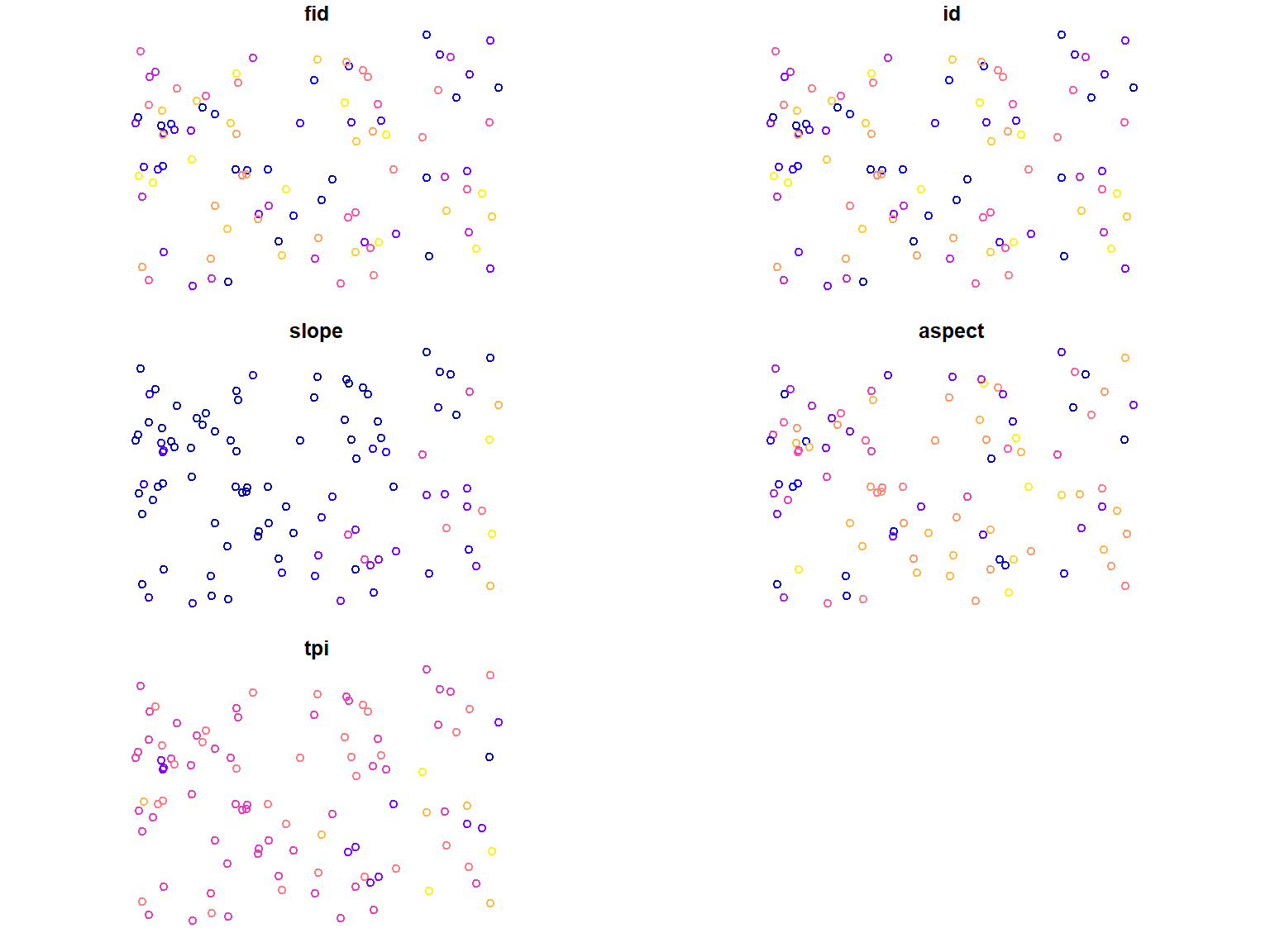# .shp Files

If your data is already “spatial” like .shp or .gpkg files, we do not have to transform them into a spatial object in R. We can just check the data for layers using st_layers

``sf::st_layers("./data/derived_data/random_points.shp") ``
``````## Driver: ESRI Shapefile
## Available layers:
##      layer_name geometry_type features fields
## 1 random_points         Point      100      7``````

and read them into R using st_read. Because our object only contains a single layer, we do not have to specify it

``point_pattern_shape <- sf::st_read("./data/derived_data/random_points.shp") ``
``````## Reading layer `random_points' from data source
##   `D:\Daten\mosaicdata\spatial_data_in_R\data\derived_data\random_points.shp'
##   using driver `ESRI Shapefile'
## Simple feature collection with 100 features and 7 fields
## Geometry type: POINT
## Dimension:     XY
## Bounding box:  xmin: 26.95751 ymin: 38.96026 xmax: 27.17486 ymax: 39.0773
## Geodetic CRS:  WGS 84``````

We can also check the structure

``str(point_pattern_shape) ``
``````## Classes 'sf' and 'data.frame':   100 obs. of  8 variables:
##  \$ fid     : num  1 2 3 4 5 6 7 8 9 10 ...
##  \$ id      : num  0 1 2 3 4 5 6 7 8 9 ...
##  \$ xcor    : num  27.2 27.1 27 27 27 ...
##  \$ ycor    : num  39.1 39 39 39 39 ...
##  \$ slope   : num  0.252287 0.069853 0.001385 0.091954 0.000295 ...
##  \$ aspect  : num  2.44 3.4 4.85 5.2 4.91 ...
##  \$ tpi     : num  -8.214 -3.2235 0.0225 -6.2441 -0.0705 ...
##  \$ geometry:sfc_POINT of length 100; first list element:  'XY' num  27.2 39.1
##  - attr(*, "sf_column")= chr "geometry"
##  - attr(*, "agr")= Factor w/ 3 levels "constant","aggregate",..: NA NA NA NA NA NA NA
##   ..- attr(*, "names")= chr [1:7] "fid" "id" "xcor" "ycor" ...``````

and plot the object:

``plot(point_pattern_shape) ``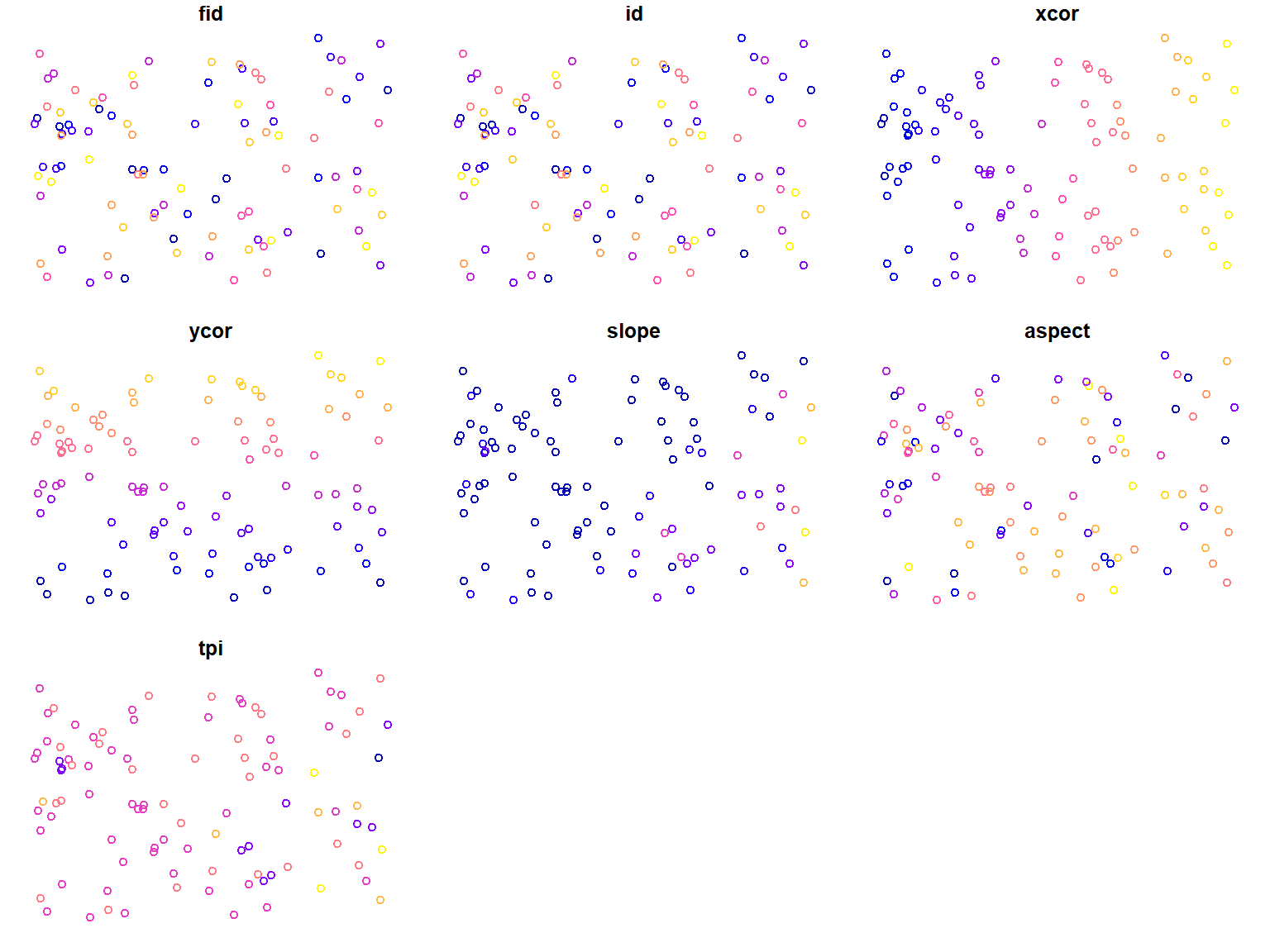# .gpkg Files

If possible, you should avoid using .shp files and use .gpkg instead. Again, we check the data for layers using st_layers

``sf::st_layers("./data/raw_data/summerschool.gpkg") ``
``````## Driver: GPKG
## Available layers:
##      layer_name geometry_type features fields
## 1    study_area       Polygon        1      0
## 2 random_points         Point      100      6
## 3       streams   Line String      137      4``````

and see, that our .gpkg consists of three layers

Therefore, we have to specify the layer we want to read in. Otherwise, the function reads the first layer

``point_pattern_gpkg <- sf::st_read("./data/derived_data/summerschool.gpkg", layer = 'random_points') ``
``````## Reading layer `random_points' from data source
##   `D:\Daten\mosaicdata\spatial_data_in_R\data\derived_data\summerschool.gpkg'
##   using driver `GPKG'
## Simple feature collection with 100 features and 6 fields
## Geometry type: POINT
## Dimension:     XY
## Bounding box:  xmin: 26.95751 ymin: 38.96026 xmax: 27.17486 ymax: 39.0773
## Geodetic CRS:  WGS 84``````

We can also check the structure

``str(point_pattern_gpkg) ``
``````## Classes 'sf' and 'data.frame':   100 obs. of  7 variables:
##  \$ id    : num  0 1 2 3 4 5 6 7 8 9 ...
##  \$ xcor  : num  27.2 27.1 27 27 27 ...
##  \$ ycor  : num  39.1 39 39 39 39 ...
##  \$ slope : num  0.252287 0.069853 0.001385 0.091954 0.000295 ...
##  \$ aspect: num  2.44 3.4 4.85 5.2 4.91 ...
##  \$ tpi   : num  -8.214 -3.2235 0.0225 -6.2441 -0.0705 ...
##  \$ geom  :sfc_POINT of length 100; first list element:  'XY' num  27.2 39.1
##  - attr(*, "sf_column")= chr "geom"
##  - attr(*, "agr")= Factor w/ 3 levels "constant","aggregate",..: NA NA NA NA NA NA
##   ..- attr(*, "names")= chr [1:6] "id" "xcor" "ycor" "slope" ...``````

and plot the object:

``plot(point_pattern_gpkg) ``

# Read Data for the Analysis

Because we want to work with the other layers of the .gpkg, we have also have to read them into R

``````streams <- sf::st_read("./data/derived_data/summerschool.gpkg", layer = 'streams')

study_area <- sf::st_read("./data/derived_data/summerschool.gpkg", layer = 'study_area') ``````

To visualize our spatial objects, we can just plot them

# Visualize the Spatial Objects

``plot(point_pattern_gpkg\$geom)``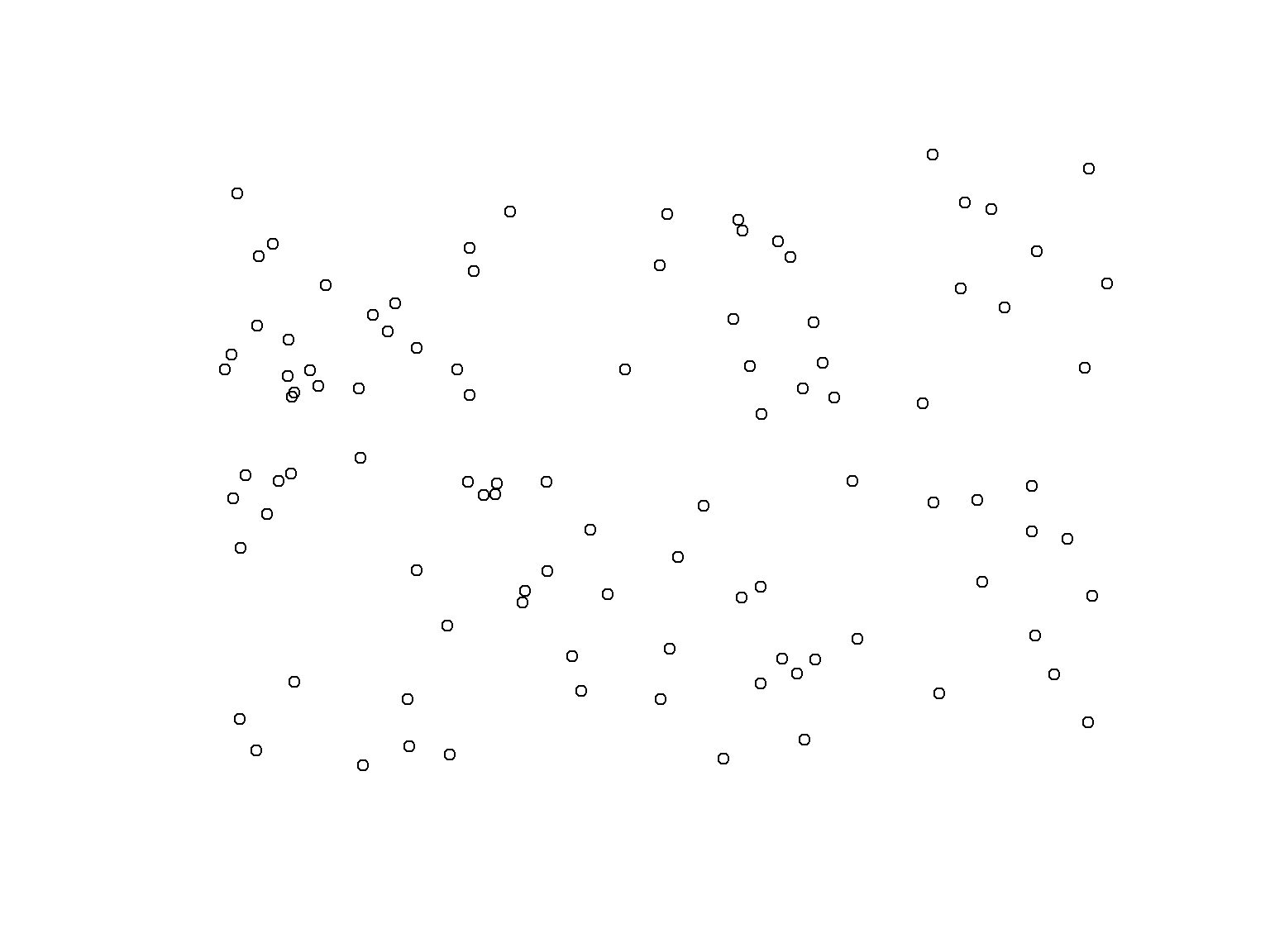# Visualize the Spatial Objects

``````plot(point_pattern_gpkg\$geom)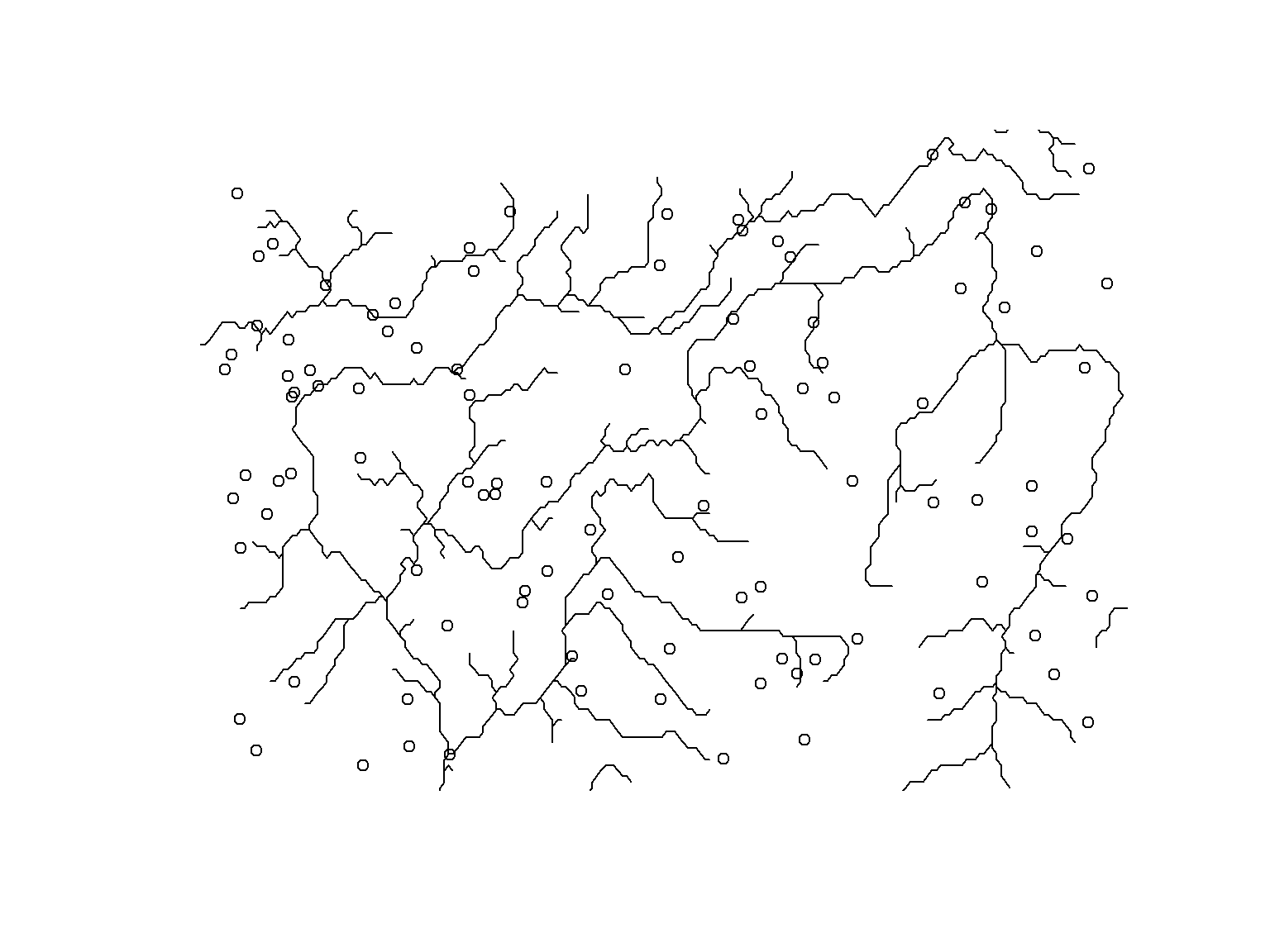# Visualize the Spatial Objects

``````plot(point_pattern_gpkg\$geom)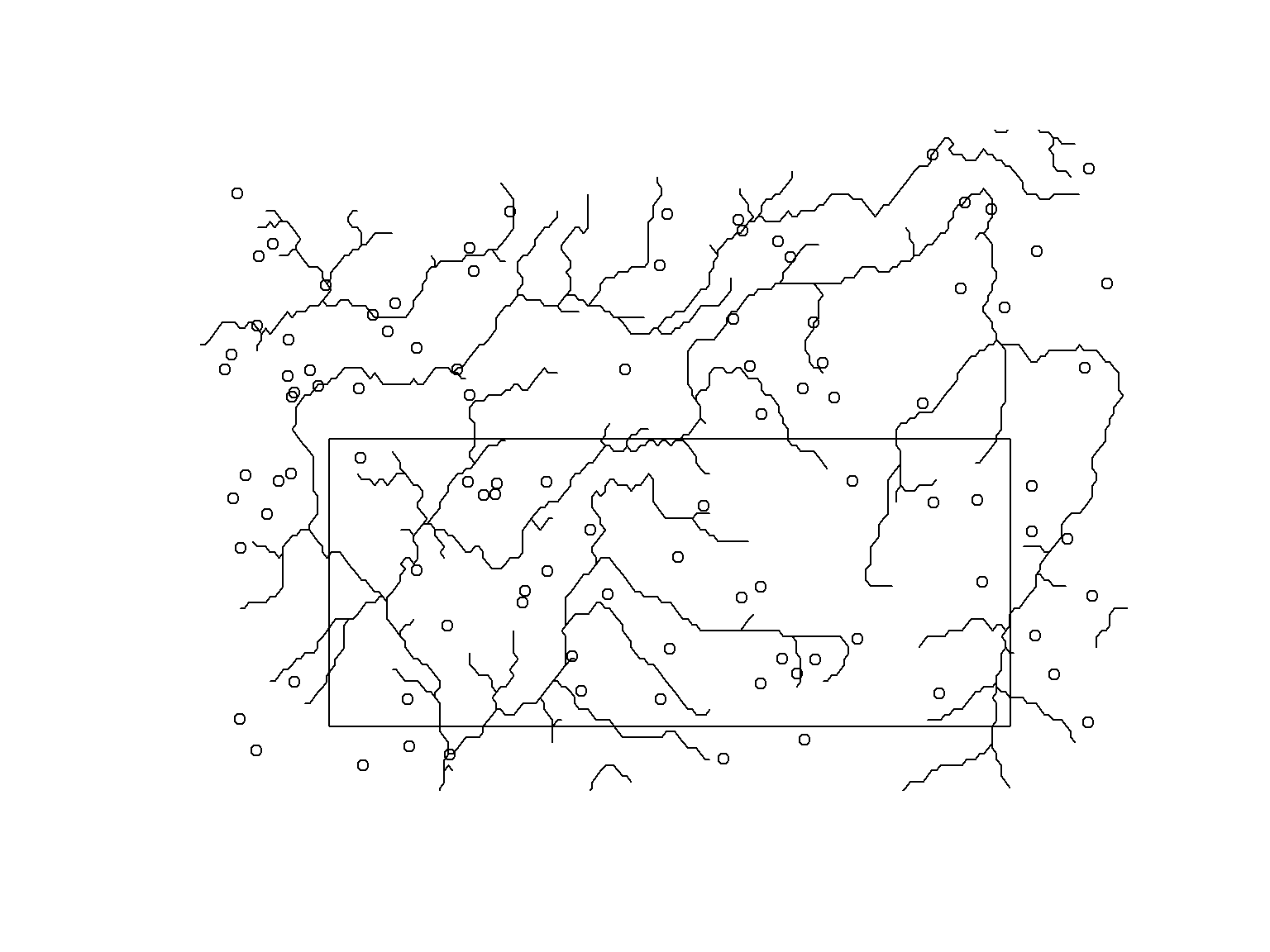# Reprojecting Vector Data

It is likely, that your data has different projections, meaning that it has different coordinate reference systems. If this is the case, we can reproject the data using the st_transform function. Again, we use an EPSG code as input.

``study_area_reprojected <- sf::st_transform(study_area, crs = 32635) ``

# Reprojecting Vector Data

If we now plot the points and our study area together

``````plot(point_pattern_gpkg\$geom)we can only see the points. The reason is, that due to the different coordinate systems, the objects do not overlap anymore.

# Reprojecting Vector Data

If we want to reproject data, it makes sense to check the CRS of the point data

``sf::st_crs(point_pattern_gpkg)\$epsg``
``##  4326``

and of the study area

``sf::st_crs(study_area_reprojected)\$epsg``
``##  32635``

# Reprojecting Vector Data

Datasets must have the same CRS to work with them in a project. Therefore, we reproject one of the datasets

``point_pattern_gpkg_reprojected <- sf::st_transform(point_pattern_gpkg, crs = sf::st_crs(study_area_reprojected\$geom)) ``

Let’s check if the CRS match

``sf::st_crs(point_pattern_gpkg_reprojected)\$epsg == sf::st_crs(study_area_reprojected)\$epsg``
``##  TRUE``

# Reprojecting Vector Data

And if they overlap

``````plot(point_pattern_gpkg_reprojected\$geom)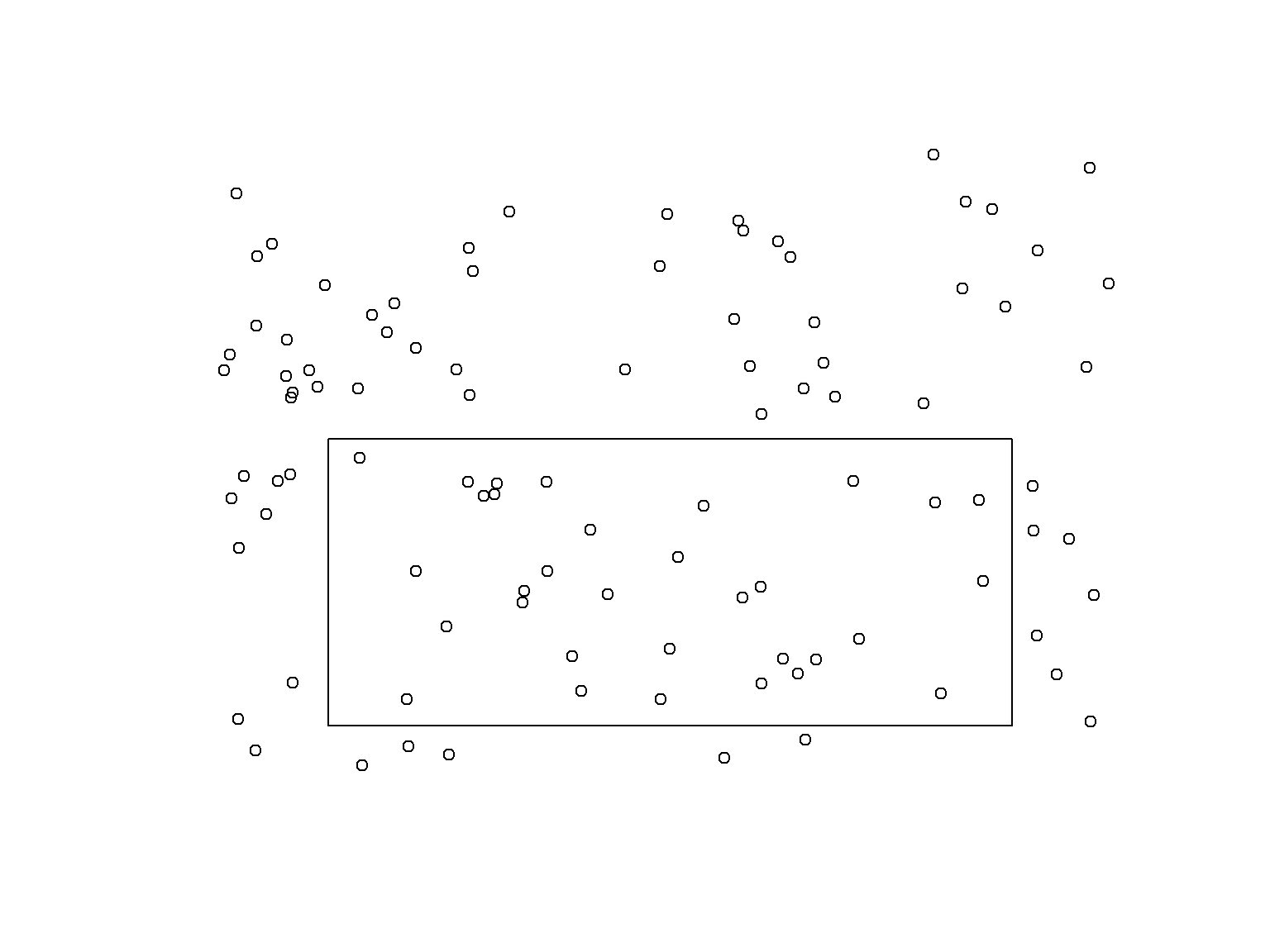And we can see that they match. Now we can work with the data.

# Working with Vector Data - Crop

On the last slides, we saw that only some of the points and the streams are in the study area (the polygon). To work only with the points in the study area, we crop the data to the extend of our study area using the st_crop function.

``````points_study_area_reprojected <- sf::st_crop(point_pattern_gpkg_reprojected, study_area_reprojected)

plot(points_study_area_reprojected\$geom)

# Working with Vector Data - Crop

And now, we crop the streams and combine the plots

``streams_study_area <- sf::st_crop(streams, study_area_reprojected) ``
``## Error in geos_op2_geom("intersection", x, y, ...): st_crs(x) == st_crs(y) is not TRUE``

# Working with Vector Data - Crop

CRS do not match. Therefore, we have to transform them first.

``streams_reprojected <- sf::st_transform(streams, crs = sf::st_crs(study_area_reprojected\$geom)) ``

# Working with Vector Data - Crop

And now, we crop the streams and combine the plots

``````streams_study_area_reprojected <- sf::st_crop(streams_reprojected, study_area_reprojected)

plot(streams_study_area_reprojected\$geom)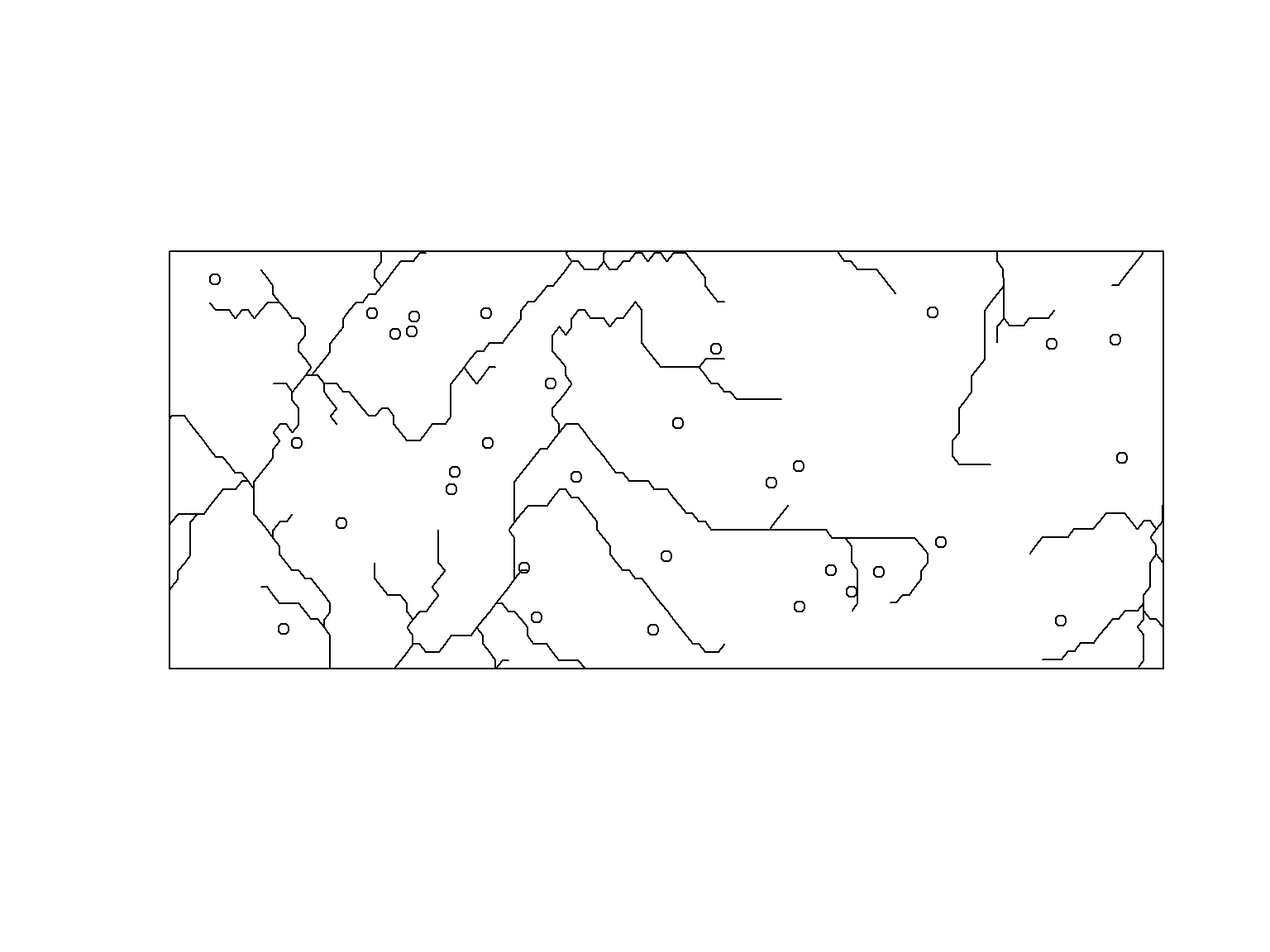# Working with Vector Data - Buffer

A buffer around vector features may also prove useful. We use the st_buffer function from the sf package to draw them. But don’t forget to check the CRS of your data, because the units for the distance of the buffer depend on it.

`` sf::st_crs(points_study_area_reprojected)\$units_gdal``
``##  "metre"``

And we can see that the unit is “metre”. But if we apply this to our ‘original’ point pattern

`` sf::st_crs(point_pattern_gpkg)\$units_gdal``
``##  "degree"``

we can see that the unit is “degree”. Measuring distances in degree is not as easy as in metres, so we stick to metres.

# Working with Vector Data - Buffer

So let’s draw a 250 m buffer aroung our points and plot them.

``````points_buffer_250 <- sf::st_buffer(points_study_area_reprojected,  250)

plot(points_buffer_250\$geom )
plot(points_study_area_reprojected\$geom, add =  TRUE, pch =  4)``````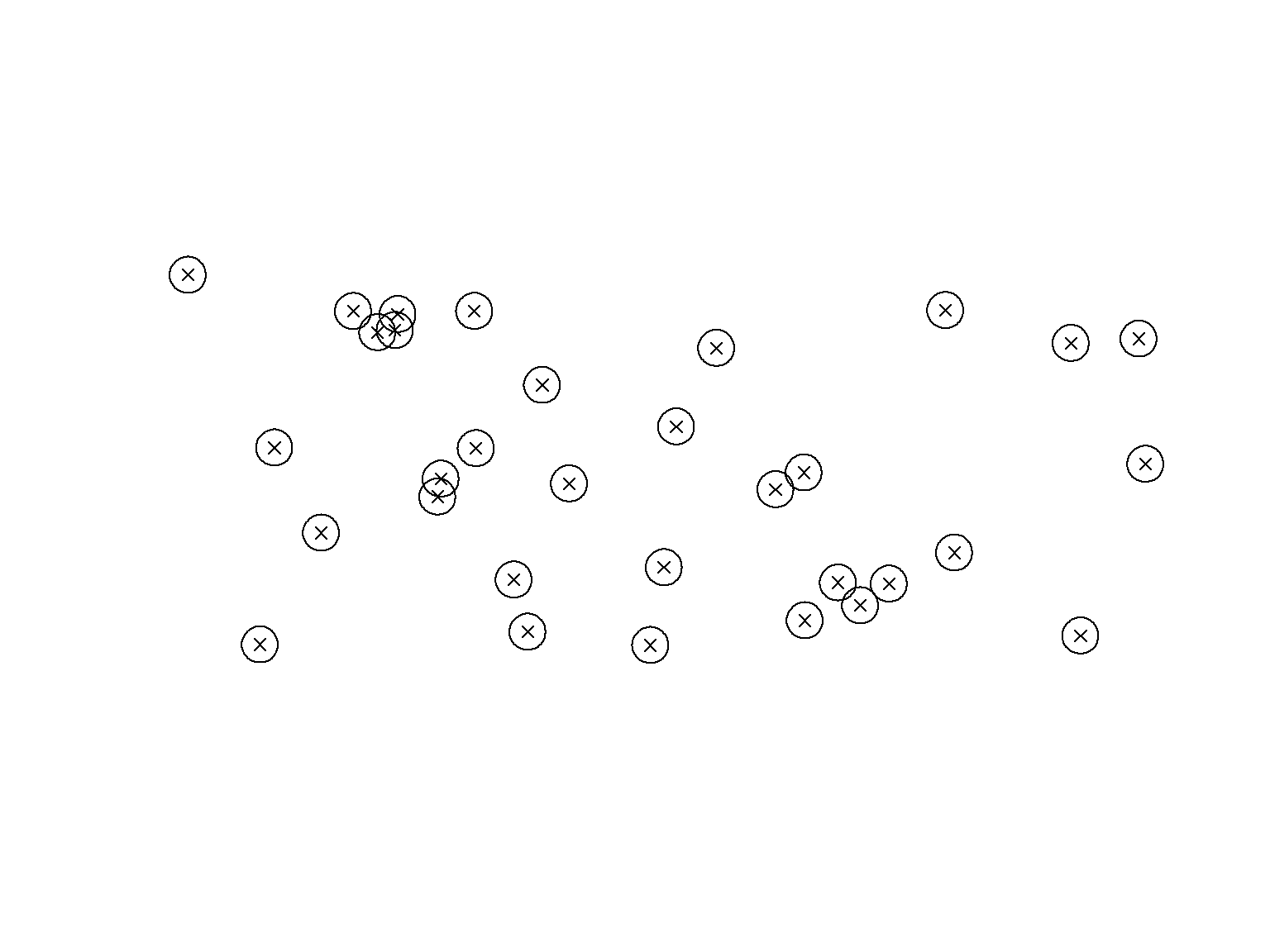# Working with Vector Data - Buffer

The same thing can be done for our streams, but with a smaller buffer.

``````streams_buffer_100 <- sf::st_buffer(streams_study_area_reprojected,  100)

plot(streams_buffer_100\$geom )
plot(streams_study_area_reprojected\$geom, add =  TRUE, lty = "dashed")``````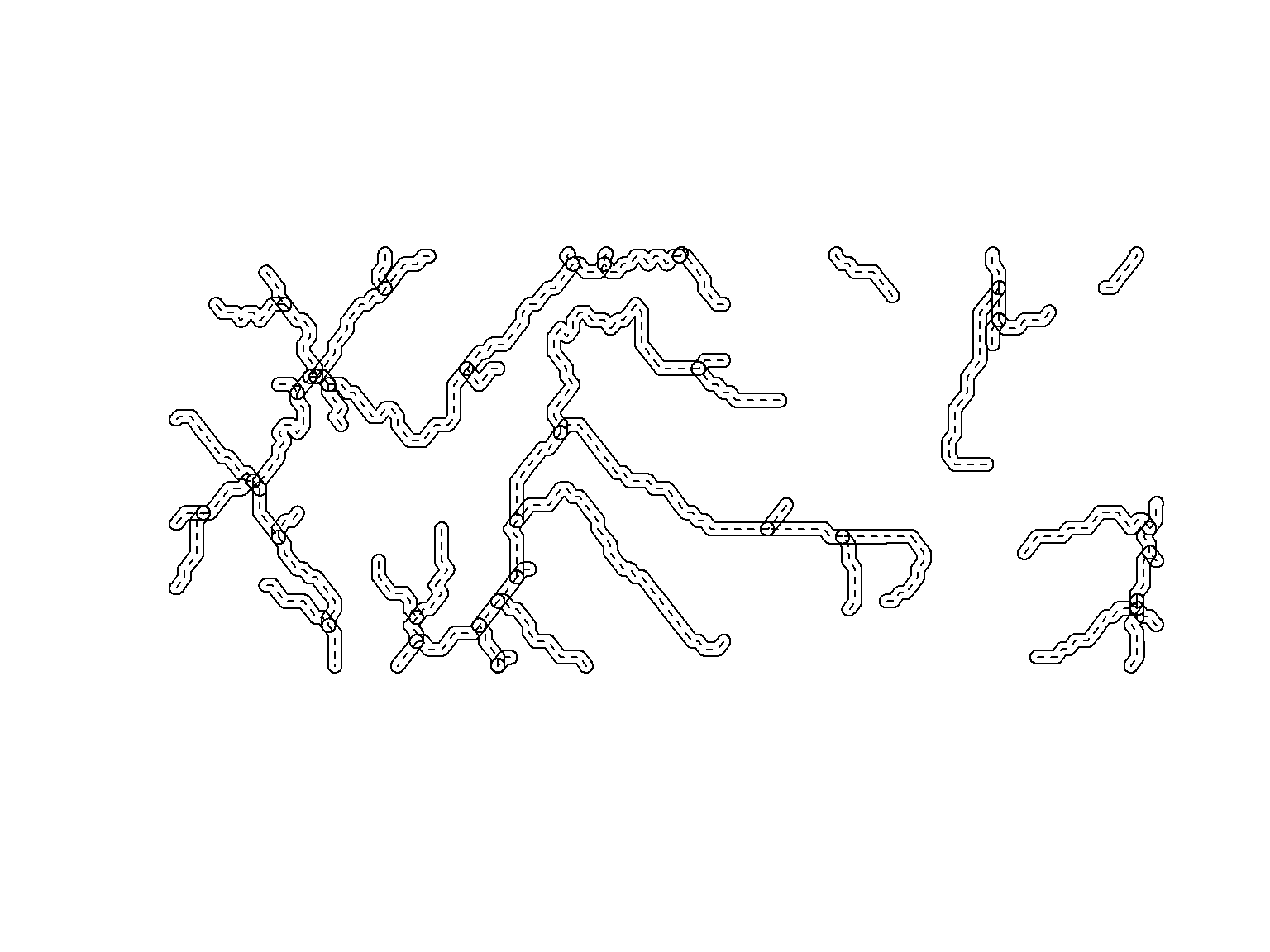# Working with Vector Data - Union

And as a last step, we unionize the our point buffers to see which of them overlap.

``````dissolved_points_buffer_250  <- sf::st_union(points_buffer_250)

plot(dissolved_points_buffer_250)
plot(points_study_area_reprojected\$ geom, add = TRUE, pch = 4)``````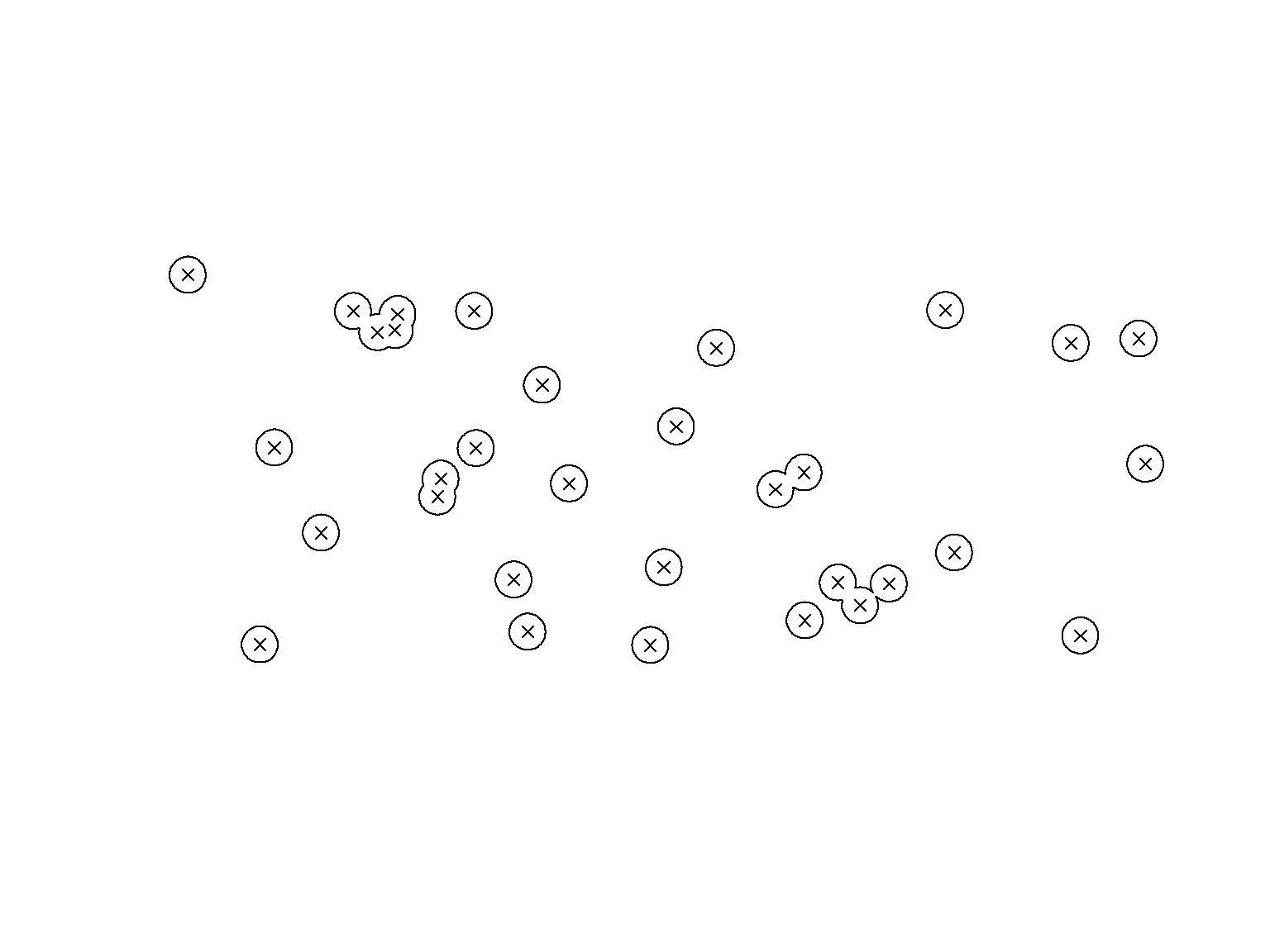# Writing Vector Data to Disk

If your analysis is finished, you can write your objects to disk by using the st_write function. If you want to work with .shp files, you just have to define a file name and a driver. If you want to update / overwrite and existing file, use the append = TRUE argument.

``````sf::st_write(study_area_reprojected,
"./data/derived_data/study_area_reprojected.shp",
driver = "ESRI Shapefile",
append = TRUE)

sf::st_write(points_study_area_reprojected,
"./data/derived_data/points_study_area_reprojected.shp",
driver = "ESRI Shapefile",
append = TRUE)

sf::st_write(streams_study_area_reprojected,
"./data/derived_data/streams_study_area_reprojected.shp",
driver = "ESRI Shapefile",
append = TRUE)``````

# Writing Vector Data to Disk

If you want to work with .gpkg files, you just have to define a layer name and use append = TRUE to add your layer to an existing .gpkg file (if you want).

``````sf::st_write(study_area_reprojected,
"./data/derived_data/summerschool_reprojected.gpkg",
layer = 'study_area_reprojected',
delete_layer = TRUE,
append = TRUE)

sf::st_write(points_study_area_reprojected,
"./data/derived_data/summerschool_reprojected.gpkg",
layer = 'points_study_area_reprojected',
delete_layer = TRUE,
append = TRUE)

sf::st_write(streams_study_area_reprojected,
"./data/derived_data/summerschool_reprojected.gpkg",
layer = 'streams_study_area_reprojected',
delete_layer = TRUE,
append = TRUE) ``````

# Working with Raster Data

In this section, we will use the terra package to work with raster data. We will look at different data formats and will work with raster data in

• the .asc and
• the .tif format.

To read raster data, we use the rast function from the terra package. At first, we use data in the .asc format

``Biomass <- terra::rast("./data/derived_data/Biomass.asc") ``

To have a look at the data, we can plot it

``terra::plot(Biomass) ``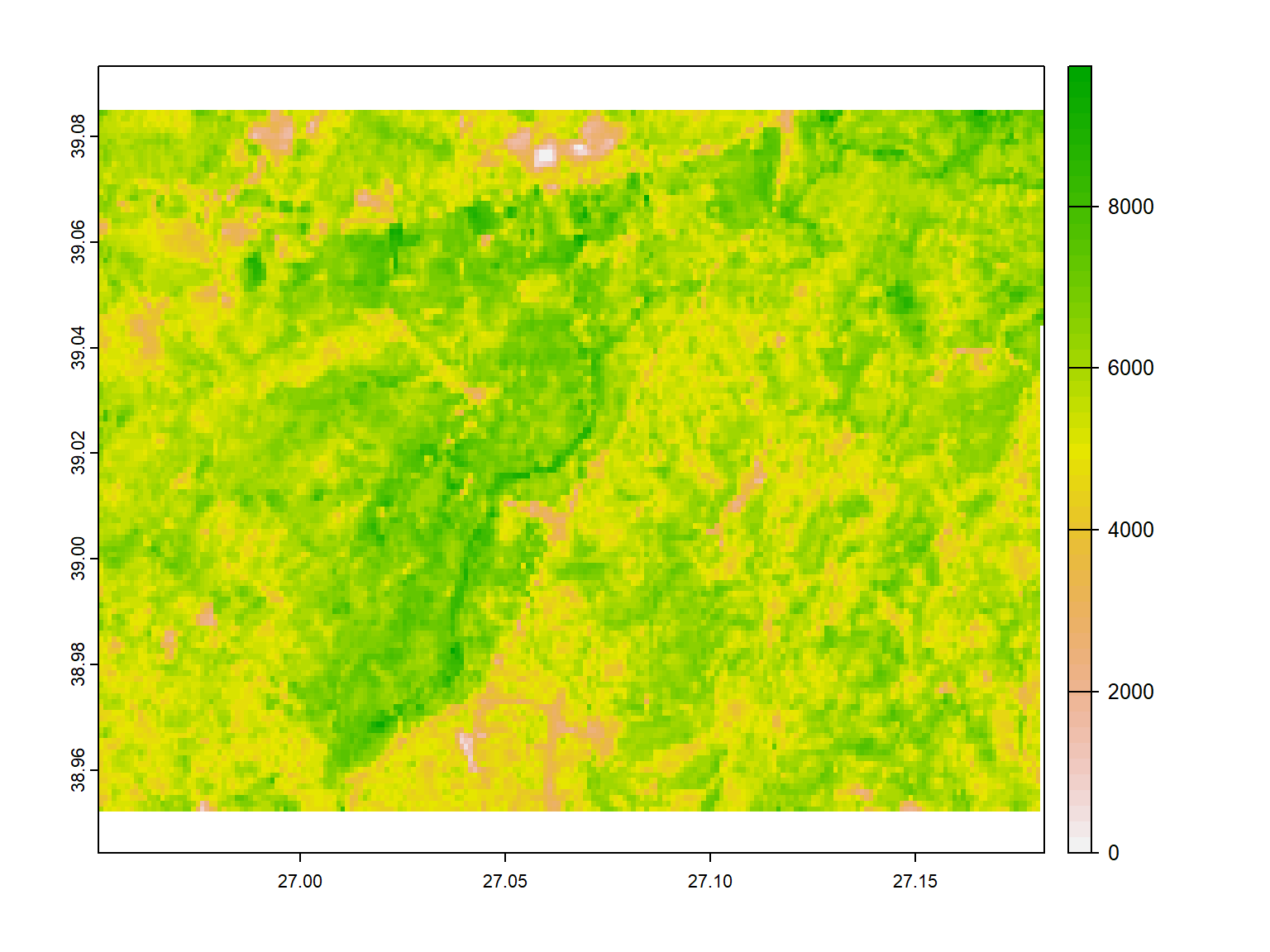Lets have a look at the structure of our loaded dataset

``Biomass``
``````## class       : SpatRaster
## dimensions  : 124, 215, 1  (nrow, ncol, nlyr)
## resolution  : 0.001072201, 0.001072201  (x, y)
## extent      : 26.95082, 27.18134, 38.95228, 39.08524  (xmin, xmax, ymin, ymax)
## coord. ref. : lon/lat WGS 84
## source      : Biomass.asc
## name        : Biomass``````

We can use the same function to read data in the .tif format

``dem <- terra::rast("./data/derived_data/dem.tif") ``

And we plot it the same way

``terra::plot(dem) ``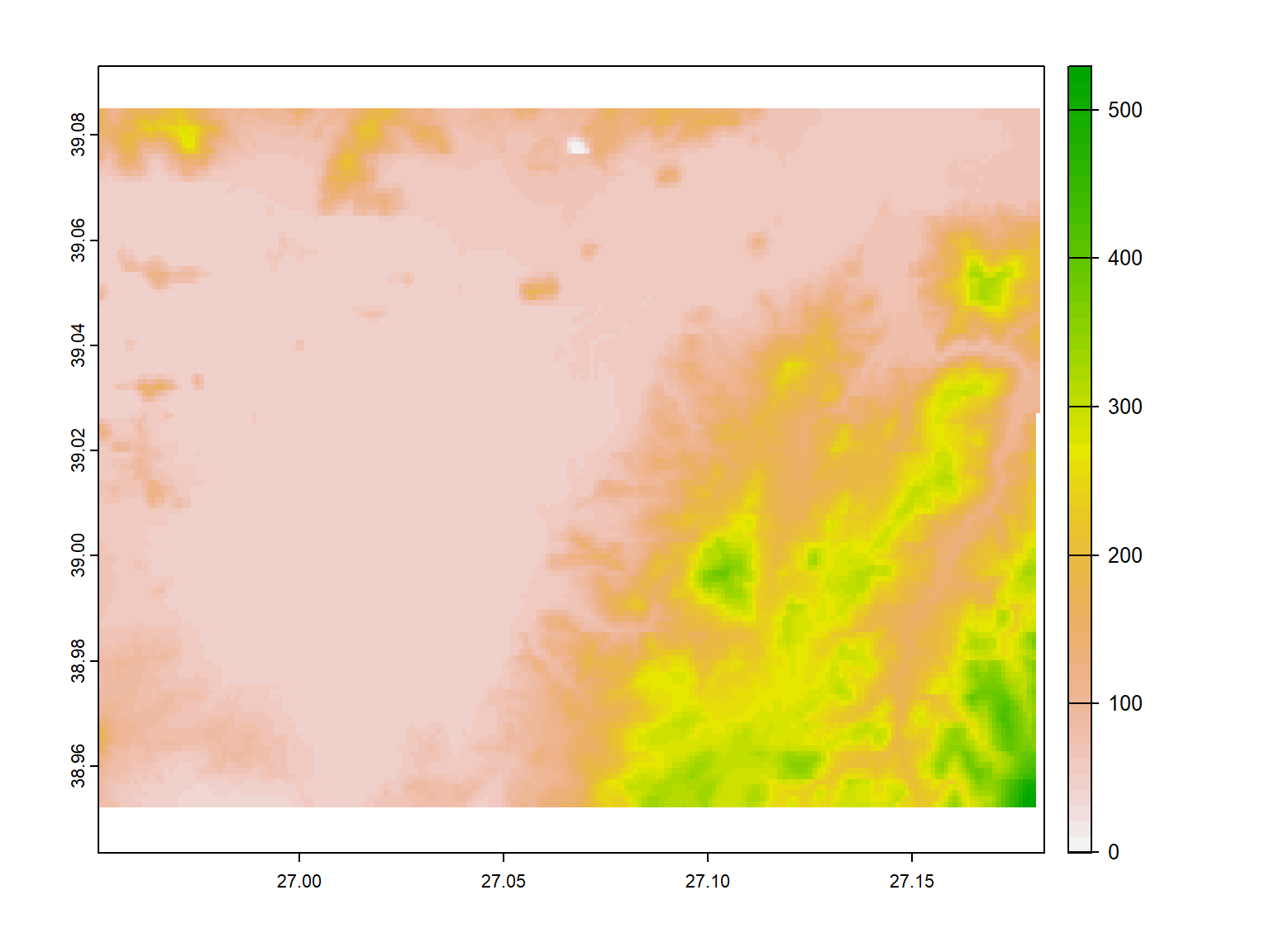Lets have a look at the structure of our loaded dataset

``dem ``
``````## class       : SpatRaster
## dimensions  : 125, 216, 1  (nrow, ncol, nlyr)
## resolution  : 0.001071998, 0.001071998  (x, y)
## extent      : 26.95082, 27.18237, 38.95124, 39.08524  (xmin, xmax, ymin, ymax)
## coord. ref. : lon/lat WGS 84 (EPSG:4326)
## source      : dem.tif
## name        :        dem
## min value   : -0.7823501
## max value   :    529.257``````

# Working with Raster Data

To work with our raster data, we use the vector data we used before, but we load it using the vect function from the terra package

``````points <- terra::vect("./data/derived_data/summerschool_reprojected.gpkg",
layer = 'points_study_area_reprojected')

streams <- terra::vect("./data/derived_data/summerschool_reprojected.gpkg",
layer = 'streams_study_area_reprojected')

study_area <- terra::vect("./data/derived_data/summerschool_reprojected.gpkg",
layer = 'study_area_reprojected') ``````

# Working with Raster Data

To look at our combined data, we plot it

``````terra::plot(dem)But the vector data doesn’t show up.

# Working with Raster Data

The reason is, that our CRS do not match.

Thus, we also reproject raster data by using the project function. Therefore, we need the EPSG information, which can be found at https://epsg.io/.

``reprojected_dem <- terra::project(dem, "epsg:32635") ``

# Working with Raster Data

To look at our combined data, we plot it

``````terra::plot(reprojected_dem)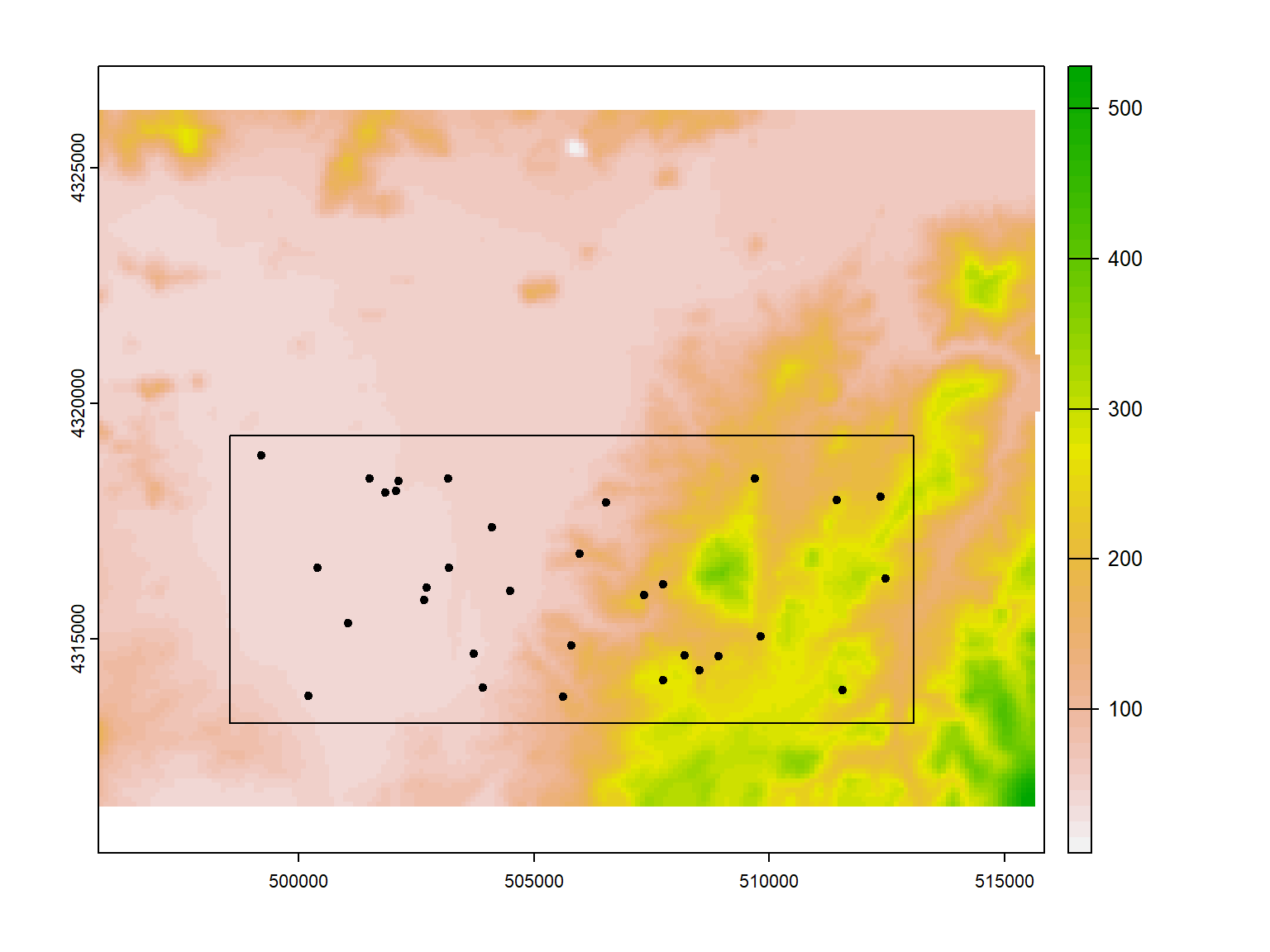# Working with Raster Data

Because we only need the elevation data in our study area, we crop the raster to the polygon and plot the results.

``````cropped_dem <- terra::crop(reprojected_dem, study_area)

terra::plot(cropped_dem)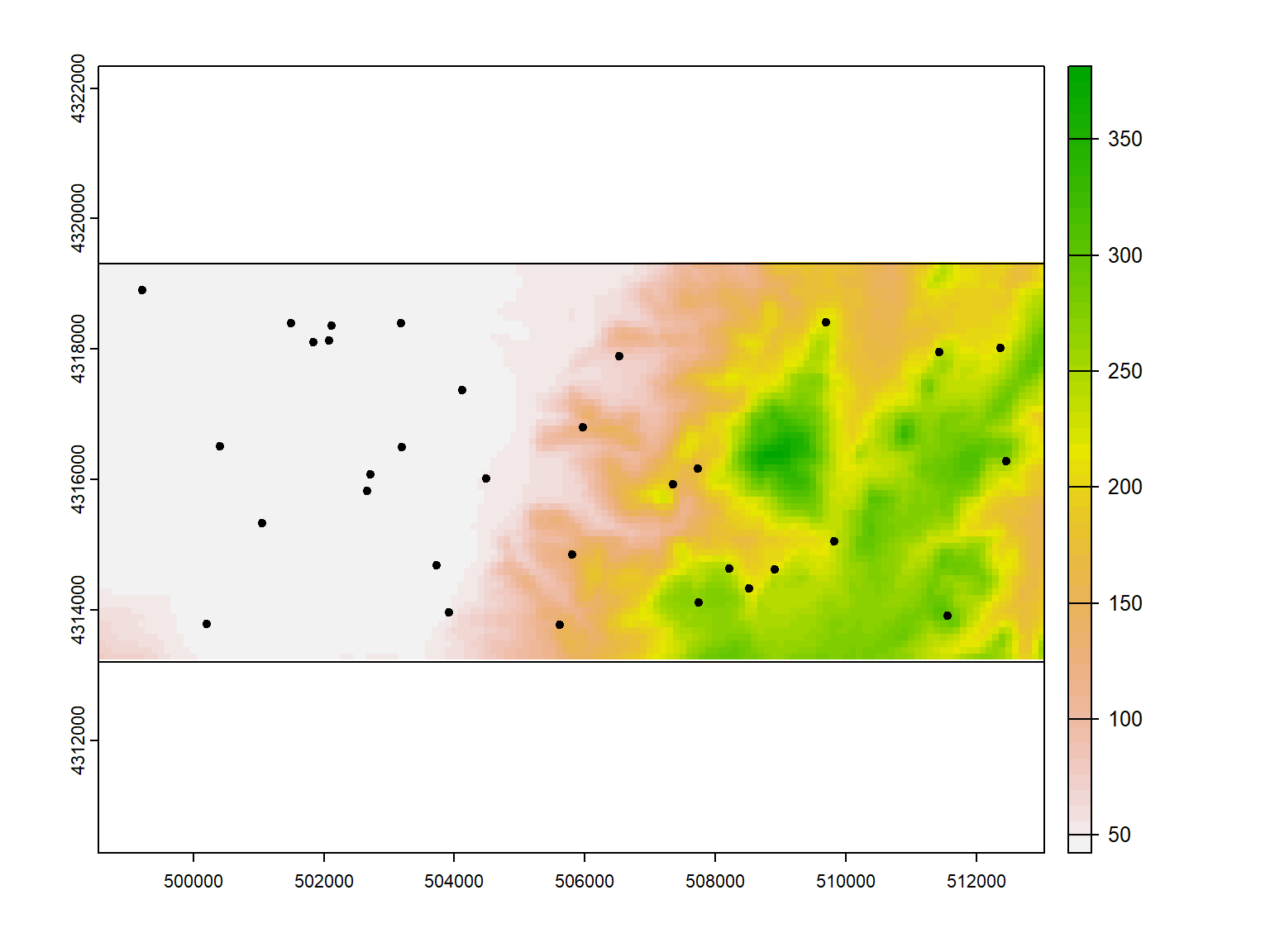# Calculate Terrrain Parameters

To calculate terrain parameters like slope or roughness from a digital elevation model, we use the terrain function from the terrain package.

To calculate the slope, we use the “slope” argument and plot the result

``````slope <- terra::terrain(cropped_dem, "slope")

terra::plot(slope) ``````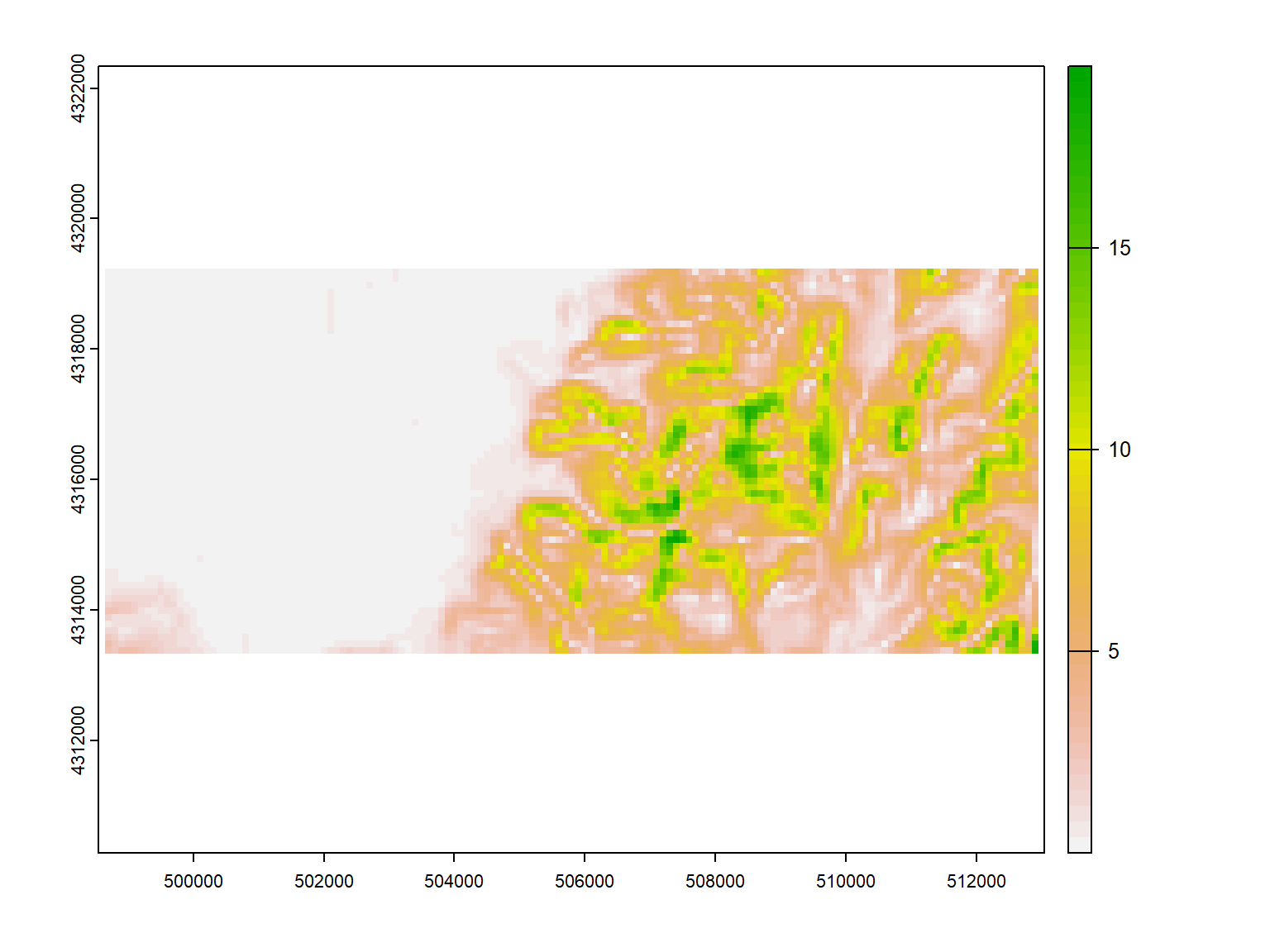# Calculate Terrrain Parameters

To calculate the roughness, we use the “roughness” argument and plot the result

``````roughness <- terra::terrain(cropped_dem, "roughness")

terra::plot(roughness) ``````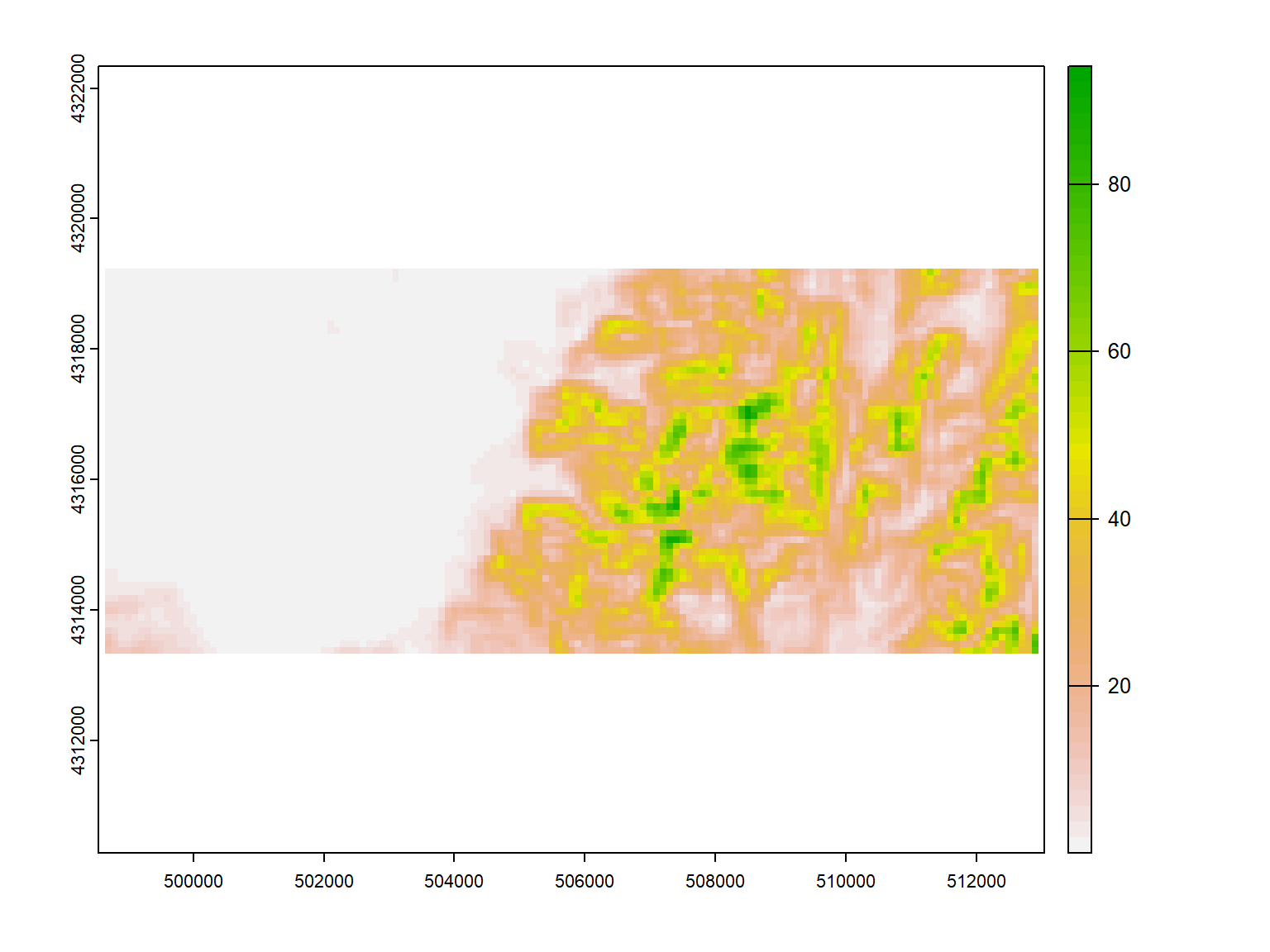# Extract Terrrain Parameters

So if we want to extract the terrain parameters at each of our points, we can use the extract function from the terra package

``points_roughness <- terra::extract(roughness,  points) ``

And let’s see what we get:

a data.frame

``points_roughness[1:5, ]``
ID roughness
1 23.8494186
2 0.1520195
3 27.2641602
4 29.8823700
5 0.3264999

# Extract Terrrain Parameters

Now we have the roughness information at each of our points and can analyse our data

``hist(points_roughness\$roughness)``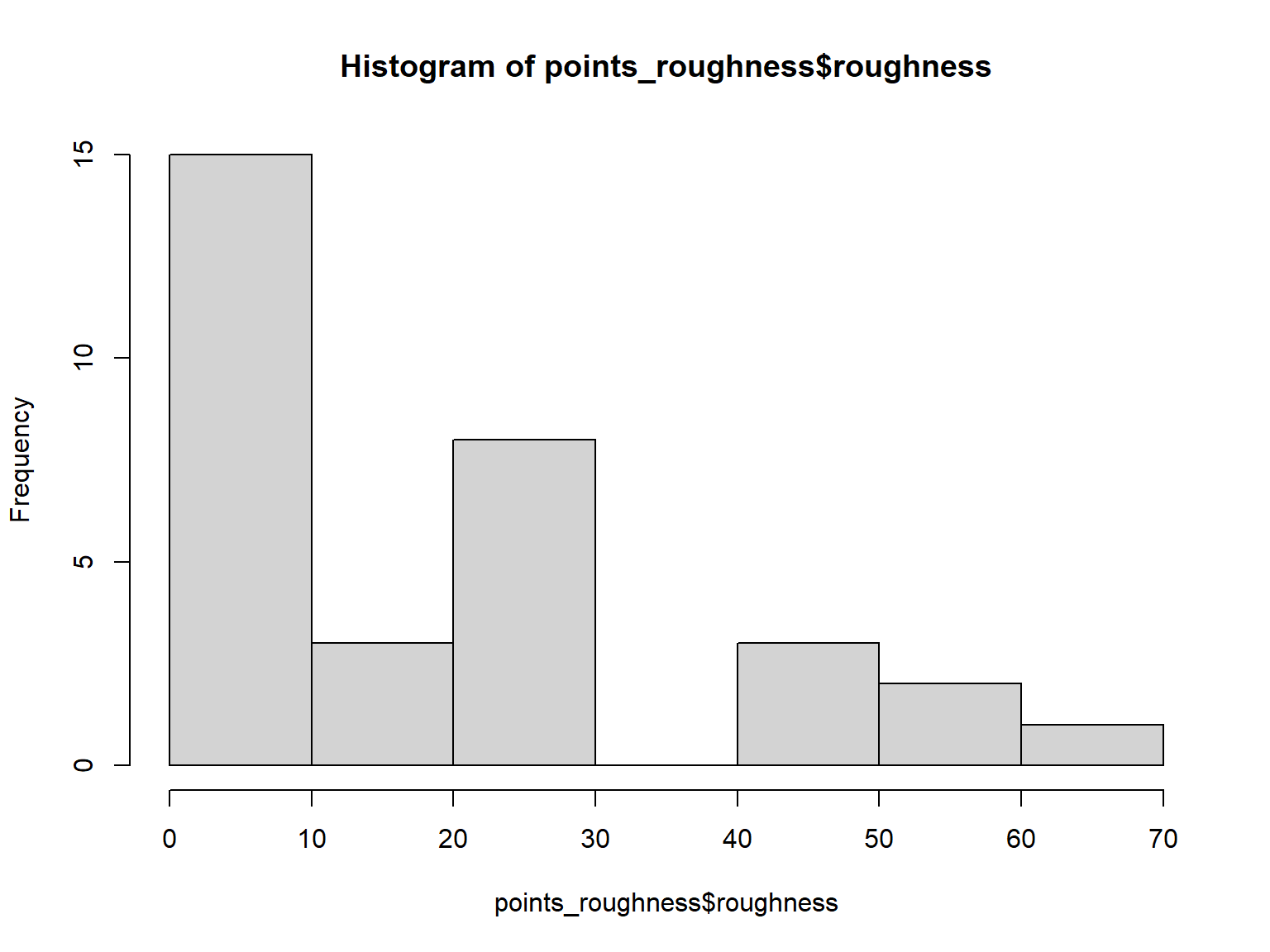and see, that most of the point are located in low roughness areas. But our data is lacking coordinates, which can be a problem for further analyses. But there is a solution

# Extract Terrrain Parameters

We have to add the xy = TRUE parameter to the function to extract the data with coordinates

``````points_roughness <- terra::extract(roughness,
points,
xy = TRUE) ``````

And let’s see what we get

# Extract Terrrain Parameters

``points_roughness[1:5, ]``
ID roughness x y
1 23.8494186 506525.9 4317885
2 0.1520195 501502.0 4318395
3 27.2641602 511563.9 4313904
4 29.8823700 505971.4 4316799
5 0.3264999 503723.6 4314679

Now we know that everything worked as expected. But let’s look at the structure of our new object

# Extract Terrrain Parameters

``str(points_roughness)``
``````## 'data.frame':    32 obs. of  4 variables:
##  \$ ID       : num  1 2 3 4 5 6 7 8 9 10 ...
##  \$ roughness: num  23.849 0.152 27.264 29.882 0.326 ...
##  \$ x        : num  506526 501502 511564 505971 503724 ...
##  \$ y        : num  4317885 4318395 4313904 4316799 4314679 ...``````

However, our new object is not a SpatialVector object, it is just a ‘data.frame’.

# Extract Terrrain Parameters

Therefore, we have to convert it into one. But if we want to work with the list, this is also possible

``````points_roughness_vect <- terra::vect(points_roughness,
geom = c("x", "y"),
crs = "epsg:32635") ``````

And now we can export our point data with the additional roughness data to our existing .gpkg

``````terra::writeVector(points_roughness_vect,
"./data/derived_data/summerschool.gpkg",
layer = 'points_roughness',
insert = TRUE,
overwrite = TRUE) ``````

# Rasterize Vector Data - Centroids inside Polygon

We can also rasterize our vector data using the rasterize function from the terra package. Here, the cropped raster is used to derive the resolution of the rasterized streams.

``streams_study_area_raster <- terra::rasterize(streams, cropped_dem) ``

And plot it

# Rasterize Vector Data - Cells touched

But if you remember, there is an additional way to rasterize vectordata, which is to use all cells touched:

``````streams_study_area_raster_touches <- terra::rasterize(streams,
cropped_dem,
touches = TRUE) ``````